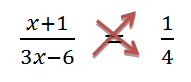# Reducing Equations To Simpler Form

Reducing equation is a method of rewriting the equation in a simpler form. Certain equations in mathematics are not in the form of linear equations but they can be put in the form of linear equations by performing some mathematical operations on them. After reduction of these equations in linear form they can be solved and the value of unknown can be calculated easily.

Let us take an example to understand the method of reducing equations in simpler form.

## Solved Examples

Example 1: Solve

$$\begin{array}{l}\frac{x+1}{3x-6}=\frac{1}{4}\end{array}$$

Solution: As the equation is not in linear form, so this cannot be directly solved for solution. Therefore first we will need to simplify the given equation by Cross Multiplication technique. In this technique, the numerator on both sides needs to be multiplied to the denominator on the other side.

After forming the Linear Equation, we can easily find the value of the unknown.

After cross multiplication, the given equation can be written as:4 (x +1) = 1 (3x – 6)

Now, using the distributive law, the parentheses can be opened as:

⇒ 4x + 4 = 3x – 6

Now using the method of transposition, the variables are brought on one side of the equation and the constants are brought on the other side.

⇒ 4x – 3x = -6 – 4

⇒ x = -10

Thus the value of the unknown in the given equation is -10.

For reducing equations to simpler form or linear form follow the following steps:

• Bring the like terms i.e. variables on one side and constants on other side of the equation
• Using the distributive law, open the brackets
• Cross multiply both sides of the equation i.e. multiply the numerator of one side by the denominator of other side
• Solve the equation as linear equation in one variable

Example 2: Solve the given linear equation:

(3x-4)/2 – (2x-1)/3 = (1/2)+x

Solution:

Given,

(3x-4)/2 – (2x-1)/3 = (1/2)+x

Taking the LCM for the left and right hand terms,

[3(3x-4)-2(2x-1)]/2.3 = (1+2x)/2

Now open the brackets to simplify the equation.

(9x-12-4x+2)/6 = (1+2x)/2

(5x-10)/6 = (1+2x)/2

Now cross-multiplying the above equation, we have

2(5x – 10) = 6(1+2x)

10x – 20 = 6 + 12x

-2x = 26

Therefore, x = -13

Test your Knowledge on Reducing Equations To Simpler Form